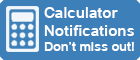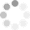Calculator Library
Free Online CalculatorsHome » 87 Calculators » Investment » Graphical Investment Calculator

Huge Domain Name Sale | Free Domains
Domain names on sale at crazy prices. Register a new domain for FREE when you buy another service.

## GRAPHICAL INVESTMENT CALCULATOR

INSTRUCTIONS

This calculator is designed to show graphically the effects of varying interest rates, time periods and deposits on your investments.

Enter your investment variables in the text boxes and click the Calculate button to calculate your result.

Results will be calculated dynamically and shown graphically as you adjust these values.

Enter Data Here

Currency:
Initial Balance:
Monthly Investment:
Interest Rate:%
Term In Years:

Initial Balance
Monthly Investments
Interest Earned
Total Value

Graphical Result#### NOTES

2. Interest is paid at the end of each year.
3. Investment balances shown are at the end of each year.
4. Interest Earned assumes interest is reinvested.
5. Total Value is the sum of your Initial Balance, Monthly Investments and Interest Earned.
6. All earnings shown are before tax.